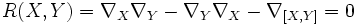# Flat connection

(diff) ← Older revision | Latest revision (diff) | Newer revision → (diff)
A connection$\nabla$ on a differential manifold$M$ is said to be flat or integrable or curvature-free or locally flat if the curvature form vanishes identically, viz for any vector fields$X$ and$Y$:$R(X,Y) = \nabla_X\nabla_Y - \nabla_Y\nabla_X - \nabla_{[X,Y]} = 0$